Laplace operator

Laplacian

The differential operatorindefined by the formula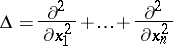(1)

(hereare coordinates in), as well as some generalizations of it. The Laplace operator (1) is the simplest elliptic differential operator of the second order. The Laplace operator plays an important role in mathematical analysis, mathematical physics and geometry (see, for example, Laplace equation; Laplace–Beltrami equation; Harmonic function; Harmonic form). Letbe an-dimensional Riemannian manifold with metric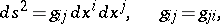(2)

let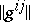be the matrix inverse to the matrix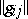and let. Then the Laplace operator (or Laplace–Beltrami operator) onwith the Riemannian metric (2) has the form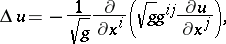(3)

where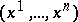are local coordinates on. (The operator (1) differs in sign from the Laplace operator onwith the standard Euclidean metric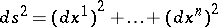.)

A generalization of the operator (3) is the Laplace operator on differential forms (cf. also Differential form). Namely, in the space of exterior differential forms onthe Laplace operator has the form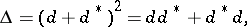(4)

whereis the operator of exterior differentiation of a form and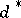is the operator formally adjoint to, defined by means of the following inner product on smooth forms with compact support:(5)

whereis the Hodge star operator induced by the metric (2) taking a-form into an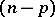-form. In (5) the formsandare assumed to be real; on complex forms one must use the Hermitian extension of the inner product (5). The restriction of the operator (4) to-forms (that is, functions) is specified by (3). On-forms with an arbitrary integer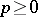the Laplace operator in local coordinates can be written in the form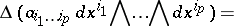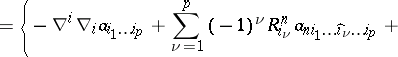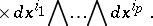Here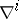and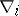are the covariant derivatives with respect to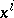(cf. Covariant derivative),is the curvature tensor and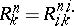is the Ricci tensor.

Suppose one is given an arbitrary elliptic complex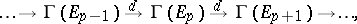(6)

where theare real or complex vector bundles onand theare their spaces of smooth sections. Introducing a Hermitian metric in each vector bundle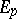and also specifying the volume element onin an arbitrary way, one can define a Hermitian inner product in the space of smooth sections ofwith compact support. Then operatorsformally adjoint to the operatorare defined. The Laplace operator (4) is then constructed on each spaceby formula (3). If for the complex (6) one takes the de Rham complex, then for a natural choice of the metric on the-forms and the volume element induced by the metric (2), one obtains for the Laplace operator of the de Rham complex the Laplace operator on forms, described above.

On a complex manifold, together with the de Rham complex there are also the elliptic complexes(7)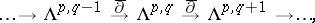(8)

where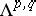is the space of smooth forms of typeon. Introducing a Hermitian structure in the tangent bundle on, one can construct the Laplace operator (4) of the de Rham complex and the Laplace operators of the complexes (7) and (8):Each of these operators takes the spaceinto itself. Ifis a Kähler manifold and the Hermitian structure onis induced by the Kähler metric, then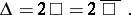An important fact, which determines the role of the Laplace operator of an elliptic complex, is the existence in the case of a compact manifoldof the orthogonal Weyl decomposition: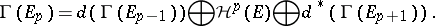(9)

In this decomposition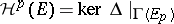, whereis the Laplace operator of the complex (6), so thatis the space of "harmonic" sections of(in the case of the de Rham complex, this is the space of all harmonic forms of degree). The direct sum of the first two terms on the right-hand side of (9) is equal to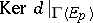, and the direct sum of the last two terms coincides with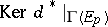. In particular, the decomposition (9) gives an isomorphism between the cohomology space of the complex (6) in the termand the space of harmonic sections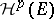.